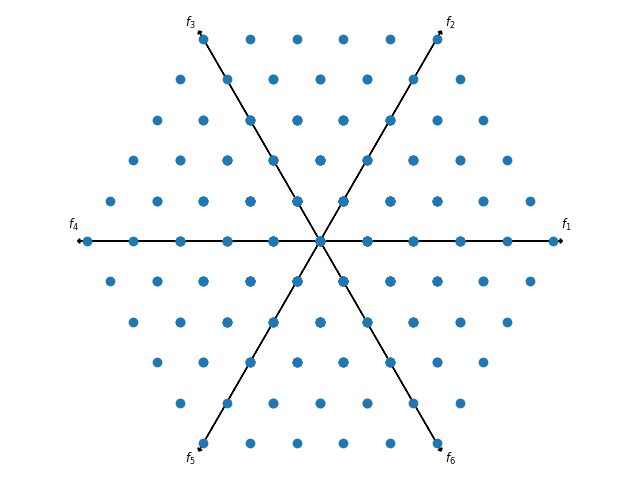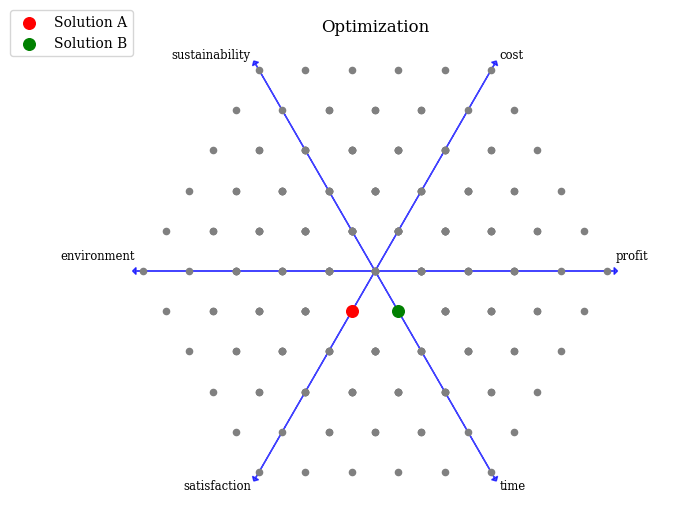Version: 0.5.0

# Star Coordinate Plot¶

Star Coordinate Plot maps a higher dimensional space with a non-linear function to two dimensions. Compared to Radviz, points can be outside of the circle.

Let us visualize some test data:

:

from pymoo.factory import get_problem, get_reference_directions

ref_dirs = get_reference_directions("uniform", 6, n_partitions=5)
F = get_problem("dtlz1").pareto_front(ref_dirs)


A simple Star Coordinate Plot plot with points can be created by:

:

from pymoo.visualization.star_coordinate import StarCoordinate


:

<pymoo.visualization.star_coordinate.StarCoordinate at 0x7f5677f17340>The plot can be further customized by supplying a title, label, and by using other plotting directives from matplotlib.
:

plot = StarCoordinate(title="Optimization",
legend=(True, {'loc': "upper left", 'bbox_to_anchor': (-0.1, 1.08, 0, 0)}),
labels=["profit", "cost", "sustainability", "environment", "satisfaction", "time"],
axis_style={"color": "blue", 'alpha': 0.7},
plot.show()

:

<pymoo.visualization.star_coordinate.StarCoordinate at 0x7f5667b99730>## API¶

class pymoo.visualization.star_coordinate.StarCoordinate(self, axis_extension=1.03, **kwargs)

Star Coordinate Plot

Parameters
axis_styledict

Most of the plots consists of an axis. The style of the axis, e.g. color, alpha, …, can be changed to further modify the plot appealing.

labelsstr or list

The labels to be used for each variable provided in the plot. If a string is used, then they will be enumerated. Otherwise, a list equal to the number of variables can be provided directly.

endpoint_styledict

Endpoints are drawn at each extreme point of an objective. This style can be modified.

Other Parameters
figsizetuple

The figure size. Default (figsize=(8, 6)). For some plots changing the size might have side-effects for position.

titlestr or tuple

The title of the figure. If some additional kwargs should be provided this can be achieved by providing a tuple (“name”, {“key” : val}).

legendstr

Whether a legend should be shown or not.

tight_layoutbool

Whether tight layout should be used.

cmapcolormap

For some plots different kind of colors are used. The colormap can be changed to modify the color sequence for the plots.